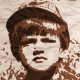# How to build a regression tree over binary variables?

Let's say I have a training set {yi,xi}N with yi>0 and xi a vector of binary variables xik∈{0,1}.

Which is the best way to build a regression tree with selected variables? Should I use CvDTree or implement my own regression tree?

edit retag close merge delete

Sort by » oldest newest most votedCvDTree is suitable for your task. It supports category (so binary too) and ordered variables, classification and regression problems. To build a tree you should use one of the CvDTree::train() methods, see doc. For example, for the first version of train method from the doc you can:

1. Load your variables to the trainData matrix of CV_32FC1 type, each row of which is a one sample variables;
2. Set tflag to CV_ROW_SAMPLE. It means that the each sample variables are located on the row in trainData.
3. Load your vector of responses yi to the 'responses' matrix of CV_32FC1 type.
4. Pass empty matrices for varIdx and sampleIdx parameters. That means that all variables and all samples will used in the training.
5. Create varType matrix of CV_8UC1 type, rows count = 1, cols count = variable_count + 1 (the last "+1" is for response type). Set CV_VAR_ORDERED (it's 0) and CV_VAR_CATEGORICAL (it's 1) for ordered and categorical variables respectively. For you task it will something like this [1,..1, 0].
6. Set empty mask for missingDataMask parameter if there are not missing values in your sample variables.
7. Fill 'params'. See doc. It's more complete than the doc for CvDTree::train().

Now you can run training a regression tree for your data.

more

Thanks a lot for your valuable suggestions and detailed descriptions. Appreciate!

I have found an example of how to use OpenCV RF for classification, which gives quite detailed explanation on how to set the params. link to code

However what I am not sure about is how these params would reflect if my problem is of regression. Can you please let me know how this can be done?

Official site

GitHub

Wiki

Documentation